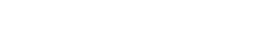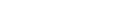1984年，冯康首次提出基于辛几何计算哈密顿体系的方法，即哈密顿体系的保结构算法，开创了哈密顿体系计算方法新领域。新的算法解决了动力学长期预测计算方法问题,促进了天体轨道、高能加速器、分子动力学等领域计算的革新。

• 1. On difference schemes and symplectic geometry

• 2. Difference schemes for Hamiltonian formalism and symplectic geometry

• 3. Symplectic geometry and numerical methods in fluid dynamics

• 4. Canonical difference schemes for Hamiltonian canonical differential equations

• 5. The symplectic methods for the computation of Hamiltonian equations

• 6. On the approximation of linear Hamiltonian systems

• 7. Construction of canonical difference schemes for Hamiltonian formalism via generating functions

• 8. Symplectic difference schemes for linear Hamiltonian canonical systems

• 9. Hamiltonian algorithms for Hamiltonian dynamical systems

• 10. The Hamiltonian way for computing Hamiltonian dynamics

• 11. Hamiltonian algorithms and a comparative numerical study

• 12. A note on conservation laws of symplectic difference schemes for Hamiltonian systems

• 13. Symplectic difference schemes for Hamiltonian systems in general symplectic structure

• 14. How to compute properly Newton's equation of motion ?

• 15. Formal power series and numerical algorithms for dynamical systems

• 16. Symplectic, contact and volume-preserving algorithms

• 17. Formal dynamical systems and numerical algorithms

• 18. Dynamical systems and geometric construction of algorithms

• 19. Variations on a theme by Euler

• 20. The step-transition operators for multi-step methods of ode's

• 21. The calculus of generating functions and the formal energy for Hamiltonian algorithms